NOTIFICATION: These examples are provided for educational purposes. The use of this code and/or information is under your own responsibility and risk. The information and/or code is given ‘as is’. I do not take responsibilities of how they are used.

Transposition Ciphers

The main idea of transposition ciphers is to rearrange the order of the letters used in the plaintext. This prevent the attacker to be able to recognise the message by using the frequency of distributions.

Rail Fence Cipher

Encryption

The basic concept of encryption on Rail Fence cipher is the follow:

1. Select a number of rows greater or equal to two. For this example, we will pick three: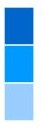2. Place each letter of the message in each row, one letter at a time, on one row at a time, from the top to the bottom
1. Lets assume the plaintext is “SUPERSECRETMESSAGE”
2. Rearrange the letters on the rows: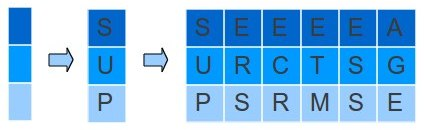3. After finished, we append one row after another in order, forming the ciphertext.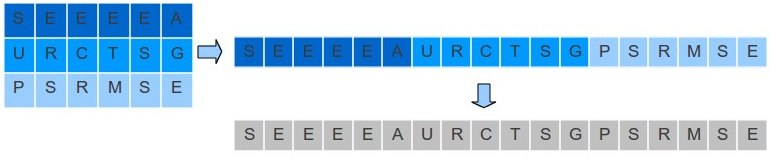Decryption

The decryption of a rail fence cipher is almost the reverse process of the encryption.

1. You will need the ciphertext and the number of rows:
1. The ciphertext is “SEEEEAURCTSGPSRMSE”
2. The number of rows is:
|rows| = 3
2. Computer the length of the ciphertext. In this case, the ciphertext “SEEEEAURCTSGPSRMSE” is:
|ciphertext| = 18
3. Lets calculate the columns that we will have:
Number of Columns = ( |ciphertext| ÷ |rows| ) + ( |ciphertext| mod |rows| )
= ( 18 ÷ 3 ) + (18 mod 3 )
= 6 + 0
= 6 columns
4. Now, we have a table of 3 rows by 6 columns:5. Let fill up this table with the ciphertext, one letter at a time, from top to down and left to right: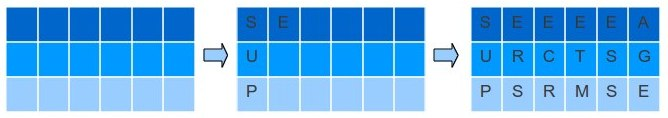6. Now recreate the plaintext from this table: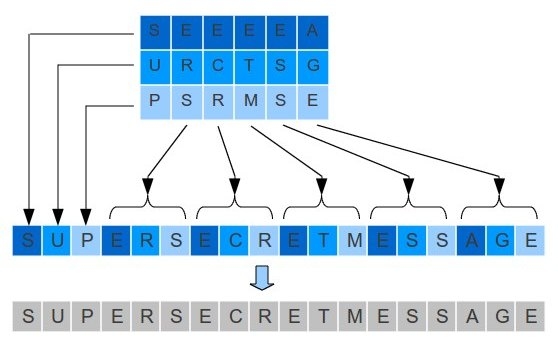vergleich.de/tagesgeld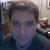Alejandro G. Carlstein Ramos Mejia